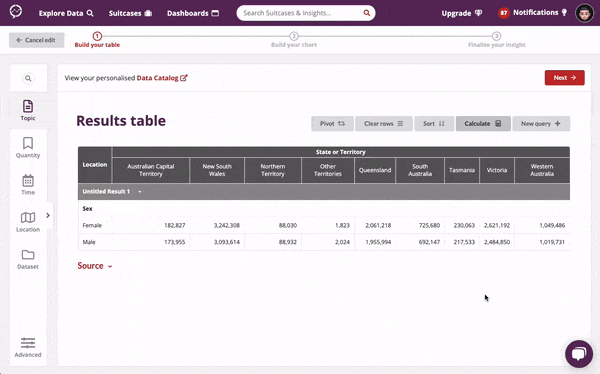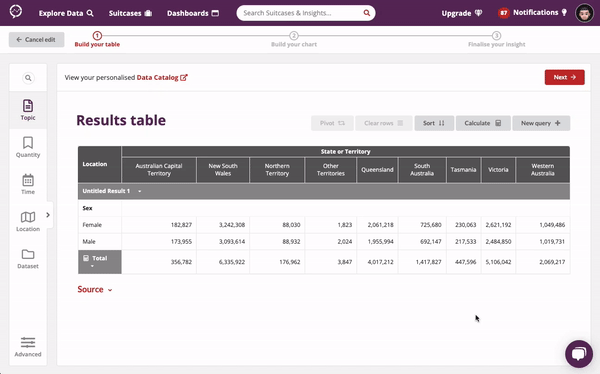1. Support Center
2. Explore Data

# How to use the Calculate Tool

## Learn how to use the Calculate Tool to add equations to your tables including totals, proportions and variances.

#### How do I create a Calculation?1. Click Calculate and the calculator will open on the left side bar.
2. Enter a formula for the calculation, noting:
1. You can do this using your mouse by clicking on the calculator buttons, or focus the formula input field and type your formula using a keyboard.
2. You can add references to rows from your insight table by clicking on the row number that will appear to the left of the table row.
1. These can also be entered manually but must be in the format Row# ; for example Row12 .
3. Hit the = button to perform the calculation and add it to the table.

#### How do I edit a Calculation?1. Click on the dropdown arrow next to the calculation row.
2. Click Edit Calculation to open the calculator on the left side bar.
3. The calculation formula will be pre-populated in the input box. Update the formula as needed and then click = to finish updating the calculation.

#### How do I rename a Calculation?

1. Click on the dropdown arrow next to the calculation row.
2. Click Rename.
3. The calculation name will become editable, update it to suit and click away from the input field to finish renaming.

#### How do I delete a Calculation?

1. Click on the dropdown arrow next to the calculation row.
2. Click Delete.

#### Formatting a Calculation

Calculations can be formatted as either a decimal or a percentage. Click on the 0.0 button to format as a decimal number (the default behaviour). Click on the % button to format as a percentage.

#### Validation

While working on a calculation you will see a green check mark to the right of the calculation formula if the formatting is valid. If the formula is invalid a red cross mark will display and the formula has to be corrected before the = button can be clicked to finalise the calculation.

#### Supported math operations

The following is a list of currently supported math symbols and operators available to the calculate tool.

• Standard arithmetic operators for addition, subtraction, division and multiplication.
• `i.e. 1+2-3/4*5`
• The power operator.
• `i.e. 5^2`
• Opening and closing Parentheses.
• `i.e. (1+5)/2`

Under the hood, calculations use a popular open source library Math.js to evaluate formulas. Beyond the supported operations listed above, there is much more functionality that can be taken advantage of (with some caveats which will be discussed).

Links to some useful Math.js functions you can make use of in the calculator:

How to use the functions:

To use the functions they must be used in the expression syntax, so for example if you wanted to get the maximum number from a set of numbers you would use the math.max function as follows:

• `max(1,3,2)+7`
• which yields 10, since 3 is the largest of the set [1,3,2] and adding 7 to it gives us 10